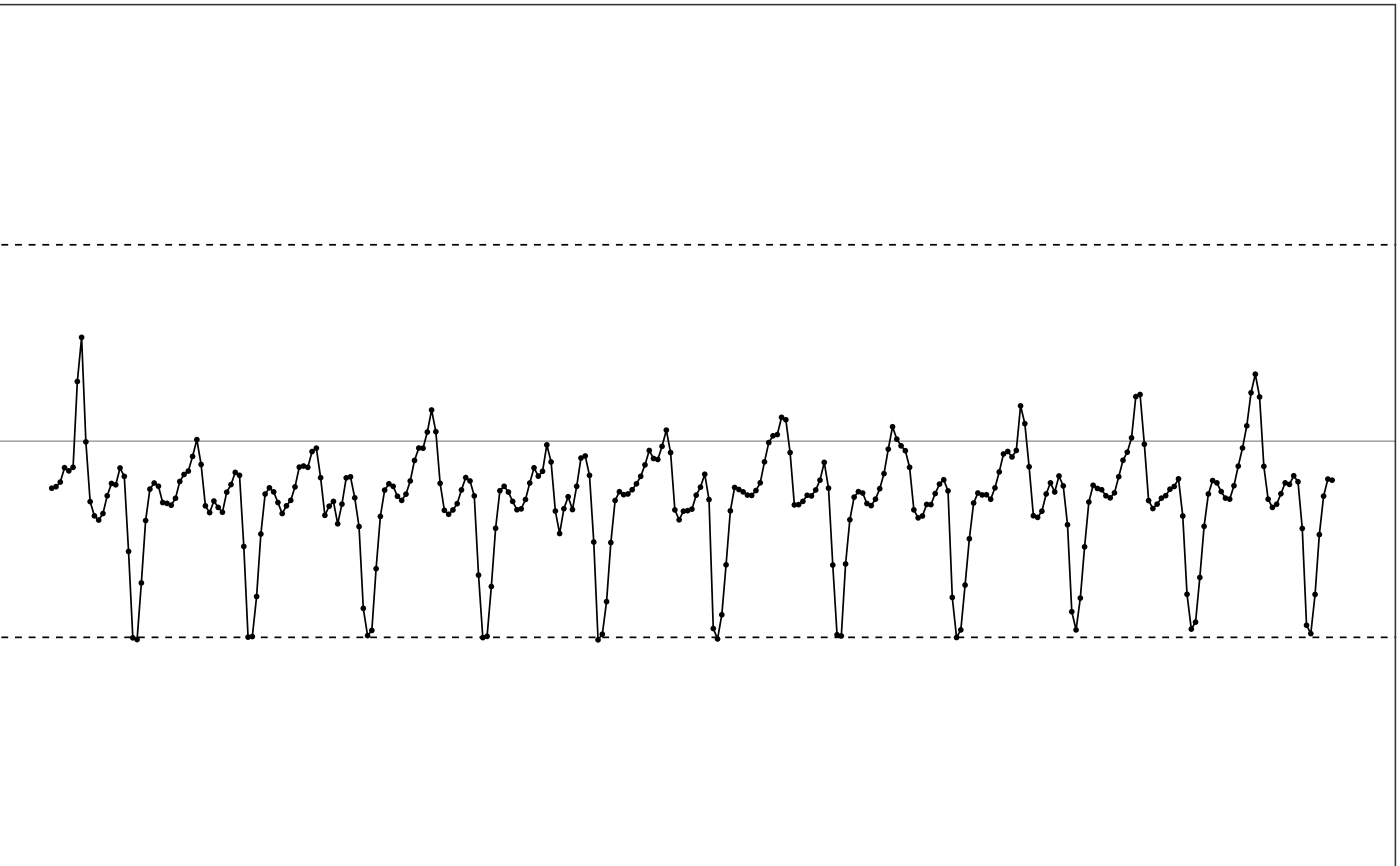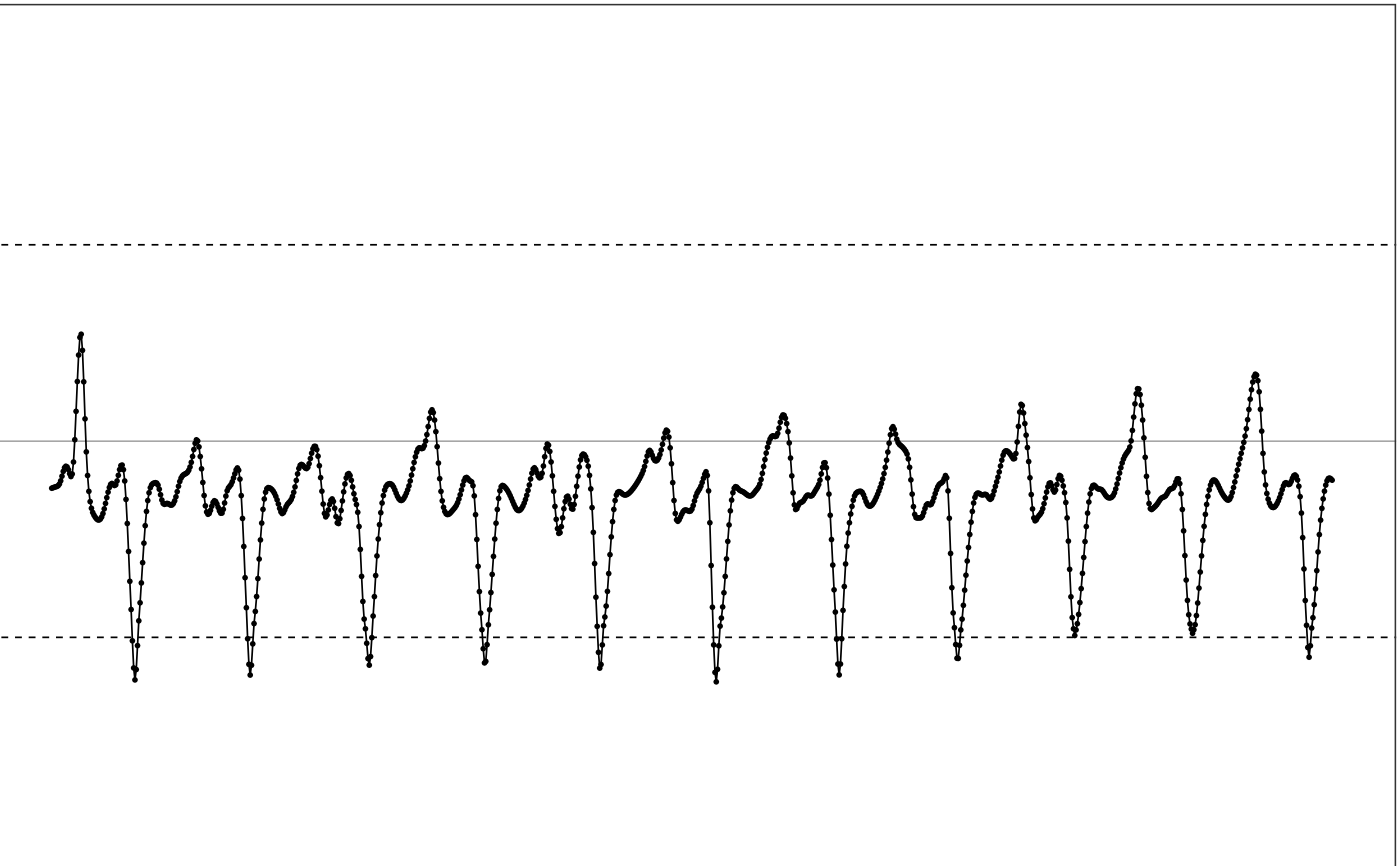extrapolate applies the extrapolation algorithm to a multi-channel accelerometer data, trying to reconstruct the true movement from the maxed-out samples.

extrapolate(df, ...)

extrapolate_single_col(
t,
value,
range,
noise_level = 0.03,
k = 0.05,
spar = 0.6
)

## Arguments

df dataframe. Input multi-channel accelerometer data. Used in extrapolate. The first column should be the date/time see following parameter list. POSIXct or numeric vector. Input index or timestamp sequence Used in extrapolate_single_col. numeric vector. Value vector used in extrapolate_single_col. numeric vector. The dynamic ranges of the input signal. Should be a 2-element numeric vector. c(low, high), where low is the negative max value the device can reach and high is the positive max value the device can reach. number. The tolerable noise level in $$g$$ unit, should be between 0 and 1. Default is 0.03, which applies to most devices. number. Duration of neighborhood to be used in local spline regression for each side, in seconds. Default is 0.05, as optimized by MIMS-unit algorithm. number. Between 0 and 1, to control how smooth we want to fit local spline regression, 0 is linear and 1 matches all local points. Default is 0.6, as optimized by MIMS-unit algorithm.

## Value

extraplate returns a dataframe with extrapolated multi-channel signal. extrapolate_single_col returns a dataframe with extrapolated single-channel signal, the timestamp col is in numeric values instead of POSIXct format.

## Details

This function first linearly interpolates the input signal to 100Hz, and then applies the extrapolation algorithm (see the manuscript) to recover the maxed-out samples. Maxed-out samples are samples that are cut off because the intensity of the underlying movement exceeds the dynamic range of the device.

extrapolate processes a dataframe of a multi-channel accelerometer signal. extrapolate_single_col processes a single-channel signal with its timestamps and values specified in the first and second arguments.

## How is it used in MIMS-unit algorithm?

This function is the first step during MIMS-unit algorithm, applied before filtering.

## See also

Other extrapolation related functions: extrapolate_rate()

## Examples

  # Use the maxed-out data for the conceptual diagram
df = conceptual_diagram_data[
conceptual_diagram_data['GRANGE'] == 4,
c("HEADER_TIME_STAMP", "X")]

# Plot input
illustrate_signal(df, range=c(-4, 4))# Use the default parameter settings as in MIMunit algorithms
# The dynamic range of the input data is -4g to 4g.
output = extrapolate(df, range=c(-4, 4))

# Plot output
illustrate_signal(output, range=c(-4, 4))